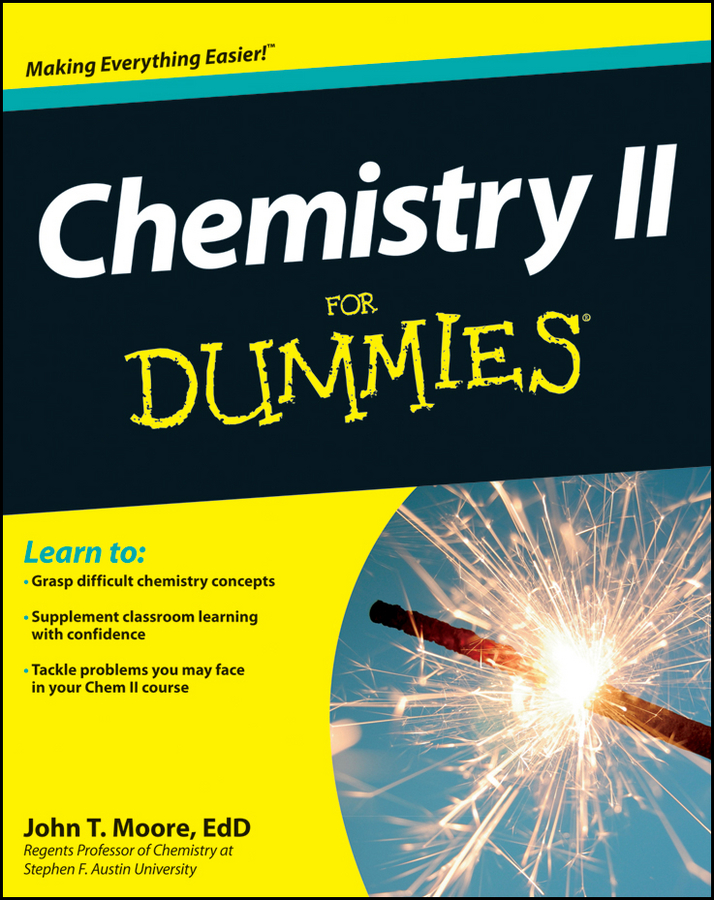# Chemistry II For Dummies

Published: 07-03-2012

The tools you need to ace your Chemisty II course

College success for virtually all science, computing, engineering, and premedical majors depends in part on passing chemistry. The skills learned in chemistry courses are applicable to a number of fields, and chemistry courses are essential to students who are studying to become nurses, doctors, pharmacists, clinical technicians, engineers, and many more among the fastest-growing professions. But if you're like a lot of students who are confused by chemistry, it can seem like a daunting task to tackle the subject. That's where Chemistry II For Dummies can help!

Here, you'll get plain-English, easy-to-understand explanations of everything you'll encounter in your Chemistry II class. Whether chemistry is your chosen area of study, a degree requirement, or an elective, you'll get the skills and confidence to score high and enhance your understanding of this often-intimidating subject. So what are you waiting for?

• Presents straightforward information on complex concepts
• Tracks to a typical Chemistry II course
• Serves as an excellent supplement to classroom learning
• Helps you understand difficult subject matter with confidence and ease

Packed with approachable information and plenty of practice opportunities, Chemistry II For Dummies is just what you need to make the grade.

## Articles From Chemistry II For Dummies

7 results
7 results
Chemistry II For Dummies Cheat Sheet

Cheat Sheet / Updated 04-08-2022

Chemistry II is more than fires and smelly explosions. Chemistry II is more about solving calculations. In fact, Chemistry II has a lot more calculations and math than your Chemistry I class did. In your Chemistry II class, you need to master several formulas so you can calculate different mathematical problems, ranging from kinetics, different types of equilibrium, thermochemistry, and electrochemistry. This Cheat Sheet can serve as a quick reference to how to solve kinetics, thermodynamics, and different types of equilibrium problems.

View Cheat Sheet
Calculating Solubility Equilibrium Problems

Article / Updated 03-26-2016

The solubility product equation is used to describe the equilibrium situation when a not-so-soluble salt is dissolving in water. For the general dissociation of a sparingly soluble salt: In this equation, x+ and z– are the magnitude of the positive and negative charge, respectively; the equilibrium constant expression (solubility product expression) is Ksp = [Mx+]a[Xz–]b

View Article
Tackling Thermodynamics Problems

Article / Updated 03-26-2016

The Gibbs Free Energy is the best indicator about whether a reaction will be spontaneous or nonspontaneous. You'll need to know this as you study Chemistry II. It has the form: In this equation ΔG° is the Gibbs Free Energy of a reaction under standard conditions of 1 atm (or 1 bar) for gases and 1 M for solutions at 25°C; ΔH° is the enthalpy of the reaction under standard conditions; T is the Kelvin temperature; and ΔS° is the entropy of the reaction under standard conditions. A spontaneous process has °G < 0. A nonspontaneous process has ΔG > 0. When °G = 0, the process is at equilibrium.

View Article
How to Solve Homogeneous Equilibrium Problems

Article / Updated 03-26-2016

The equilibrium constant describes the relationship between the amounts of the reactants and the products at a certain temperature. You'll need to know the equilibrium constant as you study Chemistry II. For the general equilibrium: the equilibrium constant expression is: In the expression, K is the equilibrium constant, the subscript c indicates this constant is expressed in terms of concentrations (not pressures, p), the brackets (as usual) stand for molar (moles/L) concentration, the uppercase letters are the reactant and product species, and the lowercase superscripts are the coefficients in the balanced chemical equation.

View Article
How to Calculate Kinetics Problems

Article / Updated 03-26-2016

The study of kinetics, the speed of chemical reactions, is essential to the study of chemistry and is a major topic in any Chemistry II class. Knowing the concepts of kinetics can help your understanding of why some reactions are fast and others slow and why some simple reactions are slow and other, more complex reactions are fast. The reaction rate (the speed of reaction) is the change in the concentration of a reactant or product per the change in time. You can write it as: Chemists normally measure concentration in terms of molarity, M, and time is usually expressed in seconds, s, which means that the units of the reaction rate are M/s. You can express the number of units in other ways such as:

View Article
Solving Acid-Base Equilibrium Problems

Article / Updated 03-26-2016

The acid and base equilibrium constant expressions describe the relationship between the amounts of reactants and products in aqueous acid-base systems. For the following general weak-acid equilibrium: the equilibrium constant expression is: For a general weak-base equilibrium: the equilibrium constant expression is: The concentration of water (or any pure liquid or solvent or solid) does appear in the equilibrium constant expression. K is the equilibrium constant, the subscript b indicates that this is an equilibrium constant expression for a weak base, and the brackets indicate molar concentrations.

View Article
Ten Great Careers in Chemistry

Article / Updated 03-26-2016